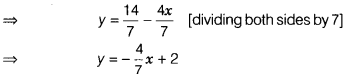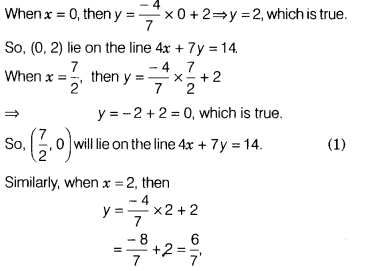# Express y in terms of x for the equation 4x + 7y = 14

Express y in terms of x for the equation 4x + 7y = 14. Check which of the points (0,2),(7/2,0) and (2,1) lie on graph of this equation

We have, 4x + 7y = 14=>7y = 14 - 4xwhich is the required form. (1)
Now, given points will lie on the graph of this equation, if they satisfy the given equation.which is not true.
So, the point (2,1) will not lie on the line 4x + 7y = 14.